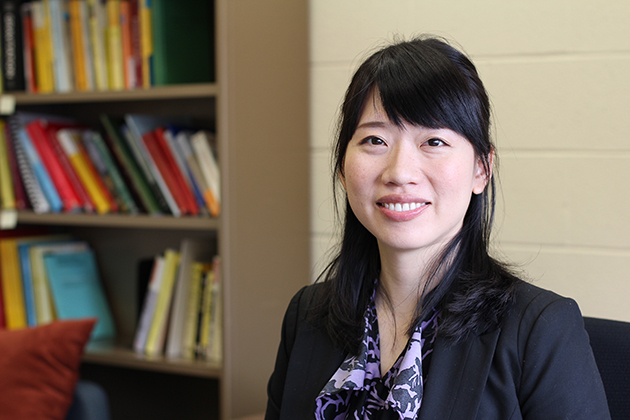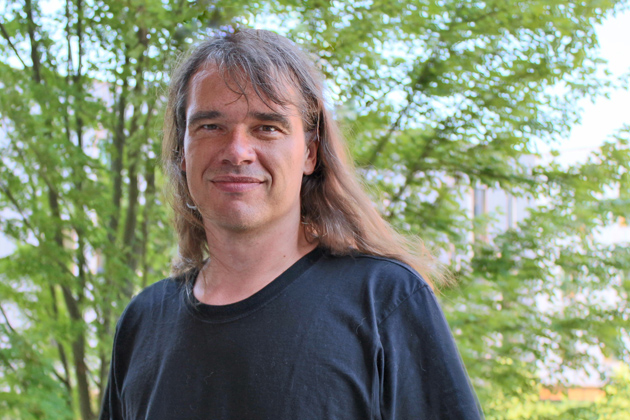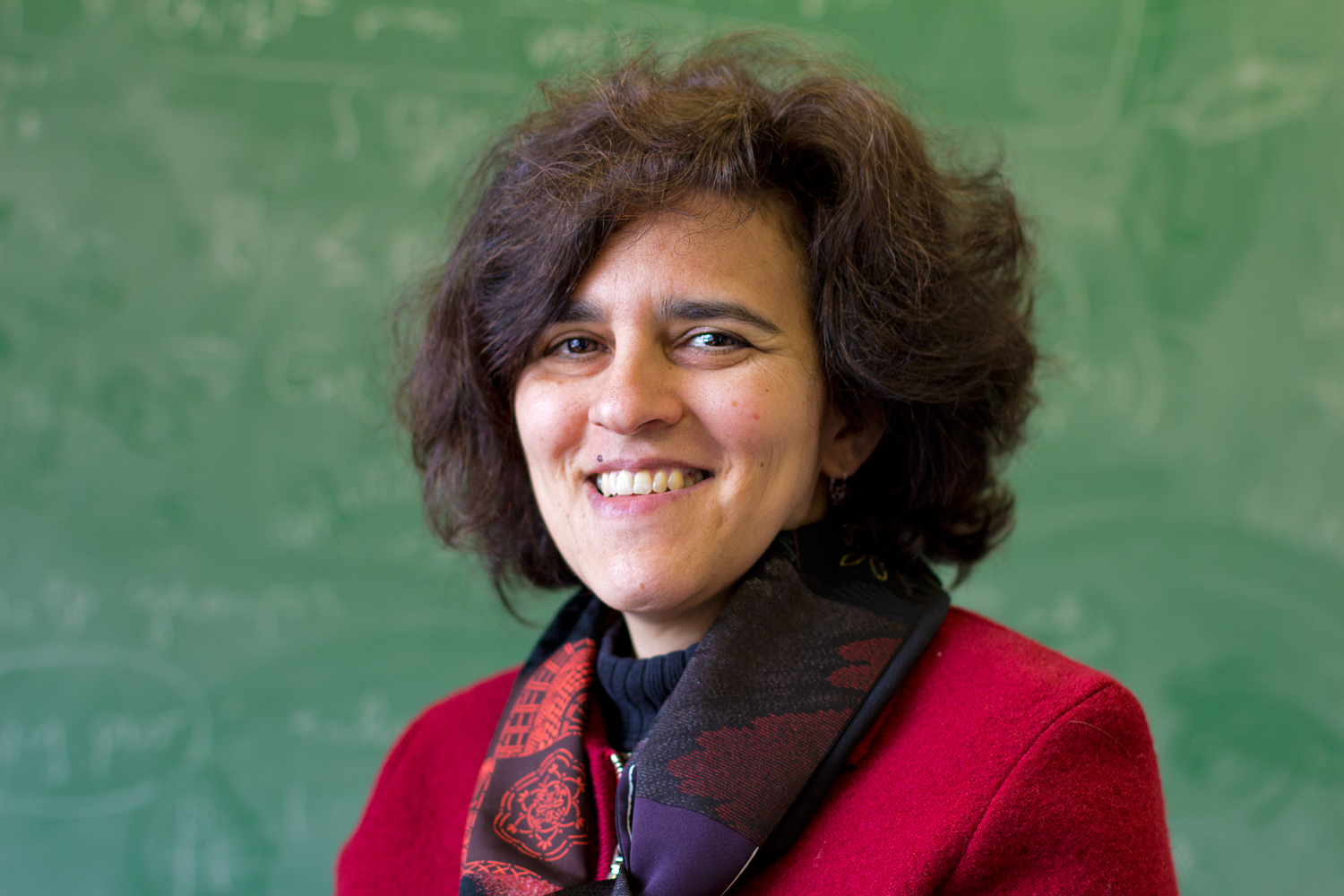# Research Spotlight## The Shape of the Universe

Understanding the shape of soap bubbles is a question that Huang, assistant professor of mathematics, says took mathematicians decades to answer definitively. In fact, the mathematician who solved the soap bubble problem won the first Fields Medal in 1936, an honor known as the Nobel Prize for mathematicians. A sphere is a very special shape, Huang says, because it has the smallest surface area containing a given volume. And because spheres minimize area, they appear more naturally in the universe.

## Cluster Algebras

Cluster algebras encompass several mathematical fields, including algebra, geometry, and combinatorics – a branch of mathematics in which researchers use a set of generators to define larger field of rational functions. More broadly, cluster algebras can be used as abstract frameworks to study other theoretical concepts in mathematics and physics. Schiffler’s work will facilitate both conceptual understanding and explicit computation of cluster algebras.## From Probability to Physics

Gordina’s research straddles the mathematics subfields of geometry, probability, and analysis. She describes it in terms of shapes, but instead of being three-dimensional, the shapes have infinite numbers of dimensions. These infinite dimensional objects exist in quantum space, so her work helps mathematicians and physicists alike to better understand the quantum theory of modern physics.

## Geometry, size, and measure

Badger’s research addresses problems related to measures, which are abstract tools used across all fields of modern mathematics to understand the concept of size. For example, measures can describe the length, area, or volume of mathematical sets drawn in two or three-dimensional Euclidean spaces. With his new project, Badger will build on his recent discoveries in geometric measures theory, the results of which may significantly increase scholars’ understanding of these essential tools.## Combined computing

We’ve come to think of computers as remarkable tools of calculation which have contributed to major scientific advances, like helping astronauts reach the moon or determining more than 31 trillion digits of Pi. But computers are not infallible; there are some functions that are simply not “computable.” This gives rise to the idea that some mathematical problems (as opposed to functions) can be solved by a computer, while others cannot. Researchers like Professor Dzhafarov are interested in the question of which are which.